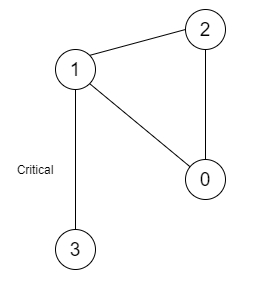# Critical Connections in a Network in C++

C++Server Side ProgrammingProgramming

Suppose there are n servers. And these are numbered from 0 to n-1 connected by an undirected server-to-server connections forming a network where connections[i] = [a,b] represents a connection between servers a and b. All servers are connected directly or through some other servers. Now, a critical connection is a connection that, if that is removed, it will make some server unable to reach some other server. We have to find all critical connections.

So, if the input is like n = 4 and connection = [[0,1],[1,2],[2,0],[1,3]],then the output will be [[1,3]]

To solve this, we will follow these steps −

• Define one set

• Define an array disc

• Define an array low

• Define one 2D array ret

• Define a function dfs(), this will take node, par, one 2D array graph,

• if node is in of visited, then −

• return

• insert node into visited

• disc[node] := time

• low[node] := time

• (increase time by 1)

• for all elements x in graph[node]

• if x is same as par, then −

• if x is not in visited, then −

• dfs(x, node, graph)

• low[node] := minimum of low[node] and low[x]

• if disc[node] < low[x], then −

• insert { node, x } at the end of ret

• Otherwise

• low[node] := minimum of low[node] and disc[x]

• From the main method, do the following −

• set size of disc as n + 1

• set size of low as n + 1

• time := 0

• Define an array of lists of size graph n + 1

• for initialize i := 0, when i < size of v, update (increase i by 1), do −

• u := v[i, 0]

• w := v[i, 1]

• insert w at the end of graph[u]

• insert u at the end of graph[w]

• dfs(0, -1, graph)

• return ret

Let us see the following implementation to get better understanding −

## Example

Live Demo

#include <bits/stdc++.h>
using namespace std;
void print_vector(vector<vector<auto> > v){
cout << "[";
for(int i = 0; i<v.size(); i++){
cout << "[";
for(int j = 0; j <v[i].size(); j++){
cout << v[i][j] << ", ";
}
cout << "],";
}
cout << "]"<<endl;
}
class Solution {
public:
set<int> visited;
vector<int> disc;
vector<int> low;
int time;
vector<vector<int> > ret;
void dfs(int node, int par, vector<int> graph[]) {
if (visited.count(node))
return;
visited.insert(node);
disc[node] = low[node] = time;
time++;
for (int x : graph[node]) {
if (x == par)
continue;
if (!visited.count(x)) {
dfs(x, node, graph);
low[node] = min(low[node], low[x]);
if (disc[node] < low[x]) {
ret.push_back({ node, x });
}
} else{
low[node] = min(low[node], disc[x]);
}
}
}
vector<vector<int> > criticalConnections(int n, vector<vector<int> >& v) {
disc.resize(n + 1);
low.resize(n + 1);
time = 0;
vector<int> graph[n + 1];
for (int i = 0; i < v.size(); i++) {
int u = v[i];
int w = v[i];
graph[u].push_back(w);
graph[w].push_back(u);
}
dfs(0, -1, graph);
return ret;
}
};
main(){
Solution ob;
vector<vector<int>> v = {{0,1},{1,2},{2,0},{1,3}};
print_vector(ob.criticalConnections(4,v));
}

## Input

4, {{0,1},{1,2},{2,0},{1,3}}

## Output

[[1, 3, ],]